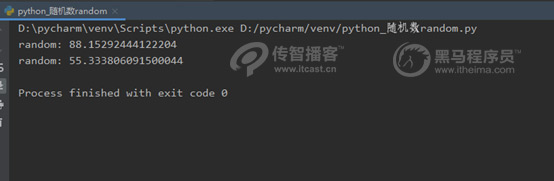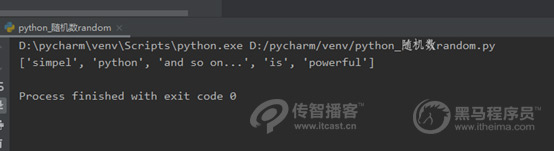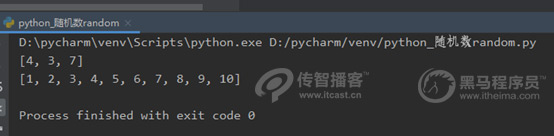# Python隨機函數random用法介紹[python培訓]

Python中的 random模塊用于生成隨機數，它提供了很多函數。接下來，針對常見的隨機數函數進行講解，具體如下

1. random.random()

import random

# 生成一個隨機數

print("random():", random.random())

# 生成第二個隨機數

print("random():", random.random())2. random.uniform(a,b)

import random

print("random:", random.uniform(50, 100))

print("random:", random.uniform(100, 50))3. random.randint(a,b)

import random

# 生成一個隨機數N，N的取值范圍為12<=N<=20

print(random.randint(12, 20))

# 生成的隨機數為N，N 的結果永遠為20

print(random.randint(20, 20))

# print(random.randint(20, 10))

# 該語句是錯誤語句，下限a必須小于上線b4、random.randrange([start], stop[, step])

import random

print(random.randrange(10, 100, 2))5. random.choice(sequence)

random.choice("學習 python")

random.choice(["JGood", , "is", "a", , "handsome", "boy"])

random.choice(("Tuple", , "List", "Dict"))

6、random.shuffle(X[,random])

import random

demo_list = ["python", "is", "powerful", "simpel", "and so on..."]

random.shuffle(demo_list)

print(demo_list)7、random.sample(squence, K)

import random

list_num =[1, 2, 3, 4, 5, 6, 7, 8, 9, 10]

# 定義slice為碎隨機在list_num中獲取的3個元素

slice = random.sample(list_num, 3)

print(slice)

# 原有的序列沒有發生改變

print(list_num)## 相關閱讀